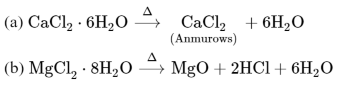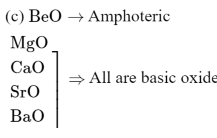# Given below are two statements:

Question:

Given below are two statements:

Statement $\mathrm{I}:$ Both $\mathrm{CaCl}_{2} \cdot 6 \mathrm{H}_{2} \mathrm{O}$ and $\mathrm{MgCl}_{2} \cdot 8 \mathrm{H}_{2} \mathrm{O}$ undergo dehydration on heating.

Statement II : $\mathrm{BeO}$ is amphoteric whereas the oxides of other elements in the same group are acidic.

In the light of the above statements, choose the correct answer from the options given below :

1. Statement I is false but statement II is true

2. Both statement I and statement II are false

3. Both statement I and statement II are true

4. Statement I is true but statement II is false

Correct Option: , 2

Solution:The dehydration of hydrated chloride of calcium can be achieved. The corresponding hydrated chloride of magnesium on heating suffer hydrolysis.